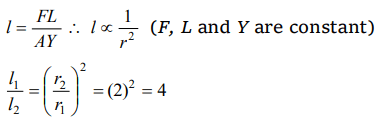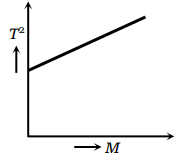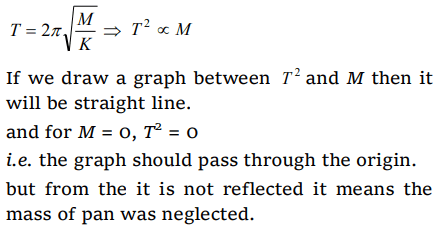## Mechanical Properties of Solids Questions and Answers Part-20

1. One end of a uniform wire of length L and of weight W is attached rigidly to a point in the roof and a weight $W_{1}$ is suspended from its lower end. If S is the area of cross-section of the wire, the stress in the wire at a height 3L/4 from its lower end is
a) $\frac{W_{1}}{S}$
b) $\frac{W_{1}+\left(W\diagup4\right)}{S}$
c) $\frac{W_{1}+\left(3W\diagup4\right)}{S}$
d) $\frac{W_{1}+W}{S}$

Explanation:2. There are two wires of same material and same length while the diameter of second wire is 2 times the diameter of first wire, then ratio of extension produced in the wires by applying same load will be
a) 1 : 1
b) 2 : 1
c) 1 : 2
d) 4 : 1

Explanation:3. A particle of mass m is under the influence of a force F which varies with the displacement x according to the relation $F=-kx+F_{0}$    in which k and $F_{0}$ are constants. The particle when disturbed will oscillate
a) about x=0,with $\omega\neq \sqrt{k\diagup m}$
b) about x=0,with $\omega= \sqrt{k\diagup m}$
c) about x= $F_{0}\diagup k$ ,with $\omega= \sqrt{k\diagup m}$
d) about x= $F_{0}\diagup k$ ,with $\omega\neq \sqrt{k\diagup m}$

Explanation:4.An elastic material of Young's modulus Y is subjected to a stress S. The elastic energy stored per unit volume of the material is
a) $\frac{2Y}{S^{2}}$
b) $\frac{S^{2}}{2Y}$
c) $\frac{S}{2Y}$
d) $\frac{S^{2}}{Y}$

Explanation: $\frac{S^{2}}{2Y}$

5.The graph shown was obtained from experimental measurements of the period of oscillations T for different masses M placed in the scale pan on the lower end of the spring balance. The most likely reason for the line not passing through the origin is that thea) Spring did not obey Hooke's Law
b) Amplitude of the oscillations was too large
c) Clock used needed regulating
d) Mass of the pan was neglected

Explanation:6. A graph is shown between stress and strain for a metal. The part in which Hooke's law holds good isa) OA
b) AB
c) BC
d) CD

Explanation: In the region OA, stress $\propto$ strain i.e. Hooke's law hold good.

7. In the above graph, point B indicates
a) Breaking point
b) Limiting point
c) Yield point
d) None of the above

Explanation: Yield point

8. In the above graph, point D indicates
a) Limiting point
b) Yield point
c) Breaking point
d) None of the above

Explanation: Breaking point

9. The strain-stress curves of three wires of different materials are shown in the figure. P, Q and R are the elastic limits of the wires. The figure shows thata) Elasticity of wire P is maximum
b) Elasticity of wire Q is maximum
c) Tensile strength of R is maximum
d) None of the above is true

Explanation: As stress is shown on x-axis and strain on yaxis10.The diagram shows a force-extension graph for a rubber band. Consider the following statementsI. It will be easier to compress this rubber than expand it
II. Rubber does not return to its original length after it is stretched
III. The rubber band will get heated if it is stretched and released
Which of these can be deduced from the graph

a) III only
b) II and III
c) I and III
d) I only